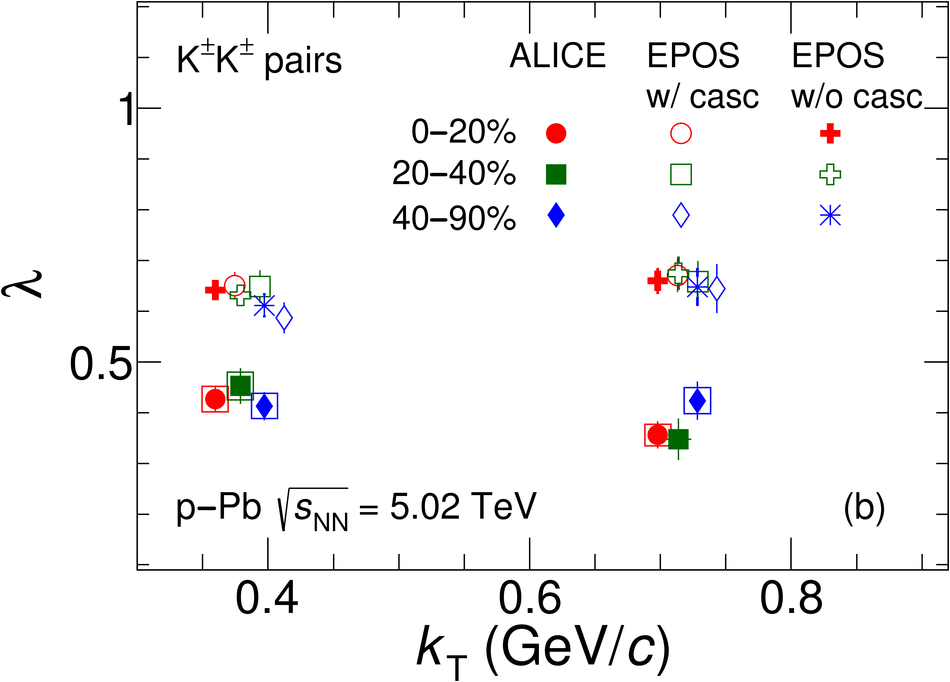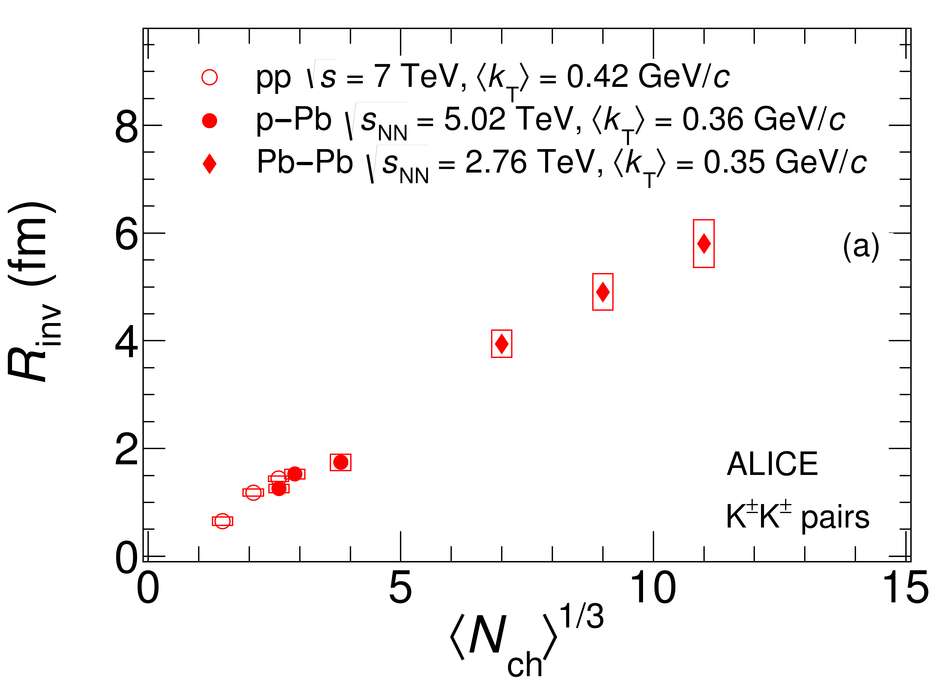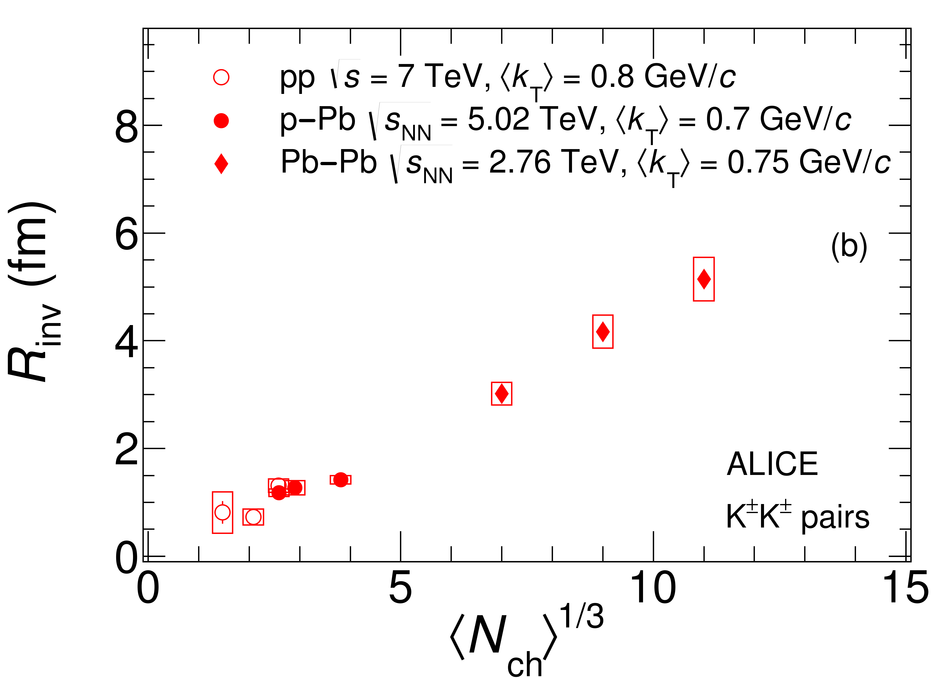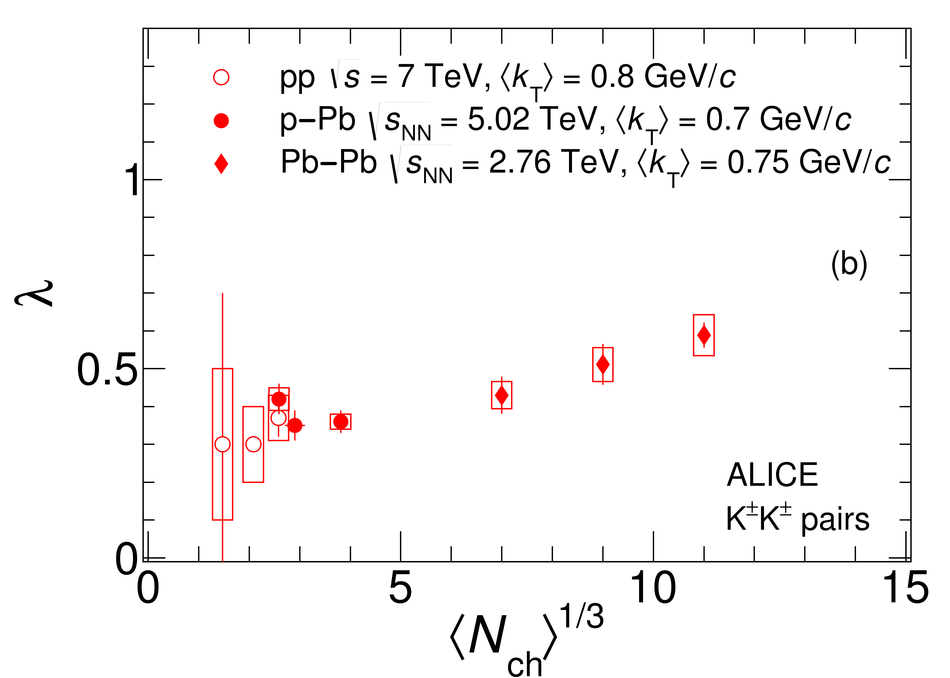# One-dimensional charged kaon femtoscopy in p-Pb collisions at $\sqrt{s_{\rm NN}}$ = 5.02 TeV

The correlations of identical charged kaons were measured in p-Pb collisions at $\sqrt{s_{\rm NN}}=5.02$ TeV by the ALICE experiment at the LHC. The femtoscopic invariant radii and correlation strengths were extracted from one-dimensional kaon correlation functions and were compared with those obtained in pp and Pb-Pb collisions at $\sqrt{s}=7$ TeV and $\sqrt{s_{\rm NN}}=2.76$ TeV, respectively. The presented results also complement the identical-pion femtoscopic data published by the ALICE collaboration. The extracted radii increase with increasing charged-particle multiplicity and decrease with increasing pair transverse momentum. At comparable multiplicities, the radii measured in p-Pb collisions are found to be close to those observed in pp collisions. The obtained femtoscopic parameters are reproduced by the EPOS 3 hadronic interaction model and disfavor models with large initial size or strong collective expansion at low multiplicities.

Figures

## Figure 1

 Single (a) and pair (b) K$^\pm$ purities for different event multiplicity classes. The systematic uncertainties associated with the purity correction are shown as boxes. Statistical uncertainties are negligible. The momentum $p$ ($k_{\rm T}$) values for lower multiplicity classes (blue and green symbols) are slightly offset for clarity.## Figure 2

 K$^\pm$K$^\pm$ experimental correlation functions corrected for purity according to Eq.~(\ref{eq:PurCorr}) (red points) and EPOS 3 model baselines (black points) versus pair relative invariant momentum $q_\mathrm{inv}$. The CFs are presented in three event multiplicity classes: 0--20\%, 20--40\% and 40--90\% and two pair transverse momentum $k_{\mathrm T}$ bins: (0.2--0.5) and (0.5--1.0)~GeV/$c$. The black line shows the fit of EPOS 3 by a first-order polynomial for $0< q_\mathrm{inv}< 1.0$~GeV/$c$. The red line shows the subsequent fit of the CF up to $q_\mathrm{inv}<$0.5~GeV/$c$ by Eq.~(\ref{eq:BS_CF}). The CFs are normalized to unity in the range $0.5< q_\mathrm{inv}< 1.0$~GeV/$c$. Statistical (lines) and systematic uncertainties (boxes) are shown.## Figure 3

 Experimental K$^\pm$K$^\pm$ invariant radii $R_\mathrm{inv}$ (a) and correlation strengths $\lambda$ (b) shown versus pair transverse momentum $k_{\mathrm T}$ for three multiplicity classes and compared with the EPOS 3 model predictions with and without the hadronic cascade phase. Statistical (lines) and systematic uncertainties (boxes) are shown. The points for lower multiplicity classes (blue and green symbols) are slightly offset in the $x$ direction for clarity.## Figure 4

 Comparison of femtoscopic radii, as a function of pair transverse momentum $k_{\rm T}$, obtained in pp and p--Pb collisions. Statistical (lines) and systematic uncertainties (boxes) are shown.## Figure 5

 Comparison of femtoscopic radii, as a function of the measured charged-particle multiplicity density $\langle N_{\rm ch}\rangle^{1/3}$, at low (a) and high (b) $k_{\rm T}$ obtained in pp , p--Pb and Pb--Pb collisions. Statistical (lines) and systematic uncertainties (boxes) are shown.## Figure 6

 Comparison of correlation strengths $\lambda$, as a function of the measured charged-particle multiplicity density $\langle N_{\rm ch}\rangle^{1/3}$, at low (a) and high (b) $k_{\rm T}$ obtained in pp , p--Pb and Pb--Pb collisions. Statistical (lines) and systematic uncertainties (boxes) are shown.## Figure 7

 The K$^{\pm}$K$^{\pm}$ correlation strengths $\lambda$ in pp , p--Pb and Pb--Pb collisions versus pair transverse momentum $k_{\rm T}$ in all multiplicity and $k_{\rm T}$ bins. Statistical (lines) and systematic uncertainties (boxes) are shown. The data points for lower multiplicity classes (blue and green symbols) are slightly offset in $k_{\rm T}$ with respect to the highest multiplicity classes (red symbols) for better visibility.